### Simultaneous Equation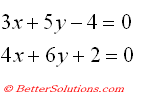Using the Solver add-in we can have more than one "Changing Cell" but we can still only have one "Set Target Cell"
A way round this is to combine the two equations to make a single equation,
Since both equations evaluate to zero the sum of the two equations must also evaluate to zero.
SS

There is a problem with using this formula though.
It is possible that the first equation might evaluate to a positive value and the second equation might evaluate to an identical negative value. In this case the equation will equal zero but the solution will not be valid.
A way round this problem is to sum the squares of the equations to avoid the possibility of them cancelling each other out.

#### Create a simple Table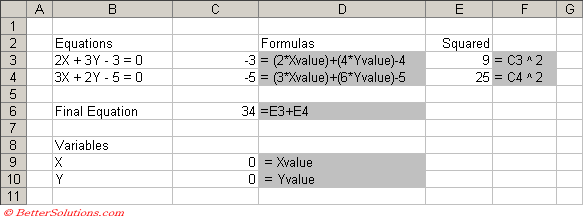Select cell "C6" and select (Tools > Solver).

You an also use the "Guess" button to let Excel guess the range of cells you want to use.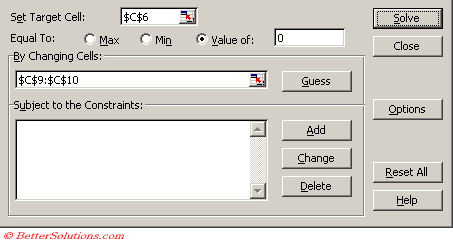Press the Solve button.

The Results dialog box will be displayed indicating if it has managed to find a solution.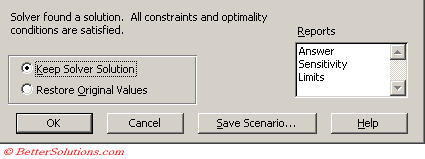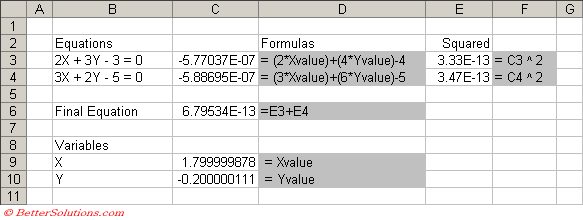If you replace the values1.8 and -0.2 into the equations you will find that they are both evaluated to zero.

#### Important

You can also use Matrix algebra to solve simultaneous equations.
If you change the Derivatives option to "Central" then Solver will obtain results which are even more accurate.